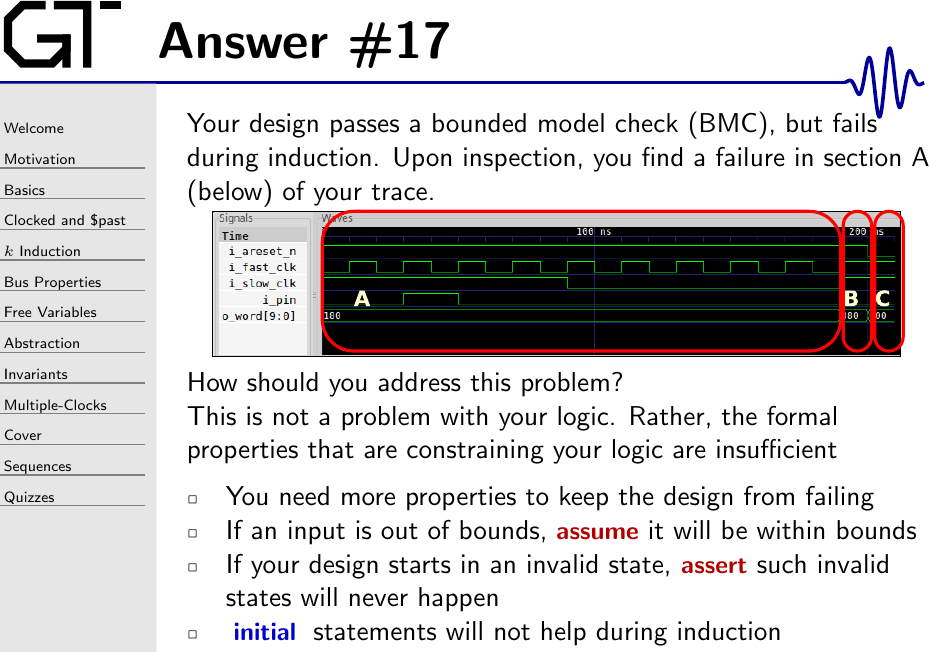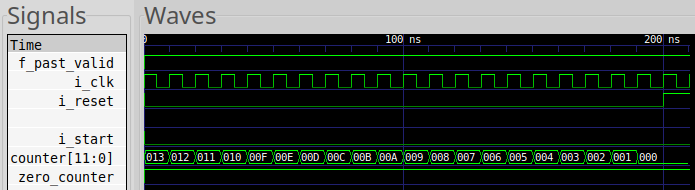Let’s illustrate this with a fun example: a counter. We’ll have this counter down from some MAX_VALUE down to zero.

We’ll also use a flag to identify every time this counter is zero.

Now, let’s make sure zero_counter is always raised when the counter finally returns to zero.

This assertion will fail induction.

Why? Because, if MAX_VALUE is longer than your induction length, then the induction engine will start from a time when counter > 0 and zero_counter=1. You’d be able to see this in section A of the trace below.This would be a fault, but it’s not the one that our property above is catching. Instead, you’d catch the fault above and then examine the formal generated trace. You’d then follow the failed assertion up stream and scratch your head wondering why zero_counter = 1 at a time when counter > 0.

The solution to this problem is to make it clear to the formal solver how counter and zero_counter are supposed to be related.

Once you do this, the problem will go away.

Note that this problem is unique to how certain solvers handle induction.